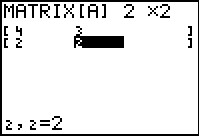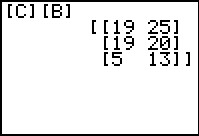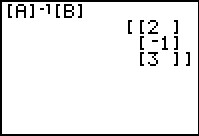# Activities

•• ##### Student Files
•• ##### Subject Area

• Math: Precalculus: Other Topics: Matrices, Sequences, and Series

• ##### Author9-12

45 Minutes

• ##### Device
• TI-83 Plus Family
• TI-84 Plus
• TI-84 Plus Silver Edition
•TI-84 Plus C Silver Edition
•TI-84 Plus CE
• ##### Report an Issue

All Systems Go!#### Activity Overview

Students learn about inverse matrices in the context of encoding/decoding secret messages and then use inverse matrices to solve systems of equations.

#### Key Steps

•In Problem 1, students use matrices to encode and decode secret messages. They do so by using the concept of an inverse matrix.

Students are asked to find the inverse of the encoding matrix and then confirm that multiplying the encoding matrix by the decoding matrix results in the identity matrix.

•The receiver of the message can retrieve the original message by decoding it using the inverse of the coding matrix.

Students will then decode the matrix on the encoded message to check that they are able to retrieve the original message.

•In Problem 2, students use inverse matrices to solve a system of equations. They multiply the inverse of the coefficient matrix by the constant matrix to obtain the solution.